Modern Physics

# Relativistic Momentum

Equation of momentum determined in Classical Mechanics

In Classical Mechanics, a body of mass m and speed v has momentum p defined by the equation shown in the table above. This definition is suitable when the modulus of v is small compared to the speed of light. However, when the velocities are “high”, in order to maintain the Principle of Conservation of Momentum, it is verified that p must be given by: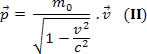In the above equation, m 0 is known as the rest mass. If we do: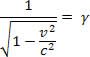The moment equation can be represented as follows: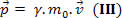In order for equation III to be equal to equation I , we can define the relativistic mass m by: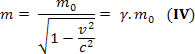Thus, the equation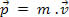represents momentum in any case, as long as m takes the value of relativistic mass. Note that if v has a much smaller value than c, we have: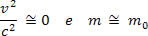In equation II, we see that as the velocity approaches c , the denominator of the fraction approaches zero and the momentum becomes infinitely large.